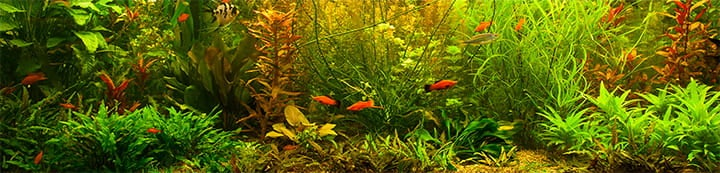1 - 5 of 5 Posts

#### jeremy1

·
##### Registered
Joined
·
379 Posts
Discussion Starter · ·
Any ideas of how I can determine the actual amount of gallons in my aquarium once I place in substrate and hardscape. I'm asking because I want to use dry ferts and I need the acutal number of gallons to properly dose correctly. Thanks

#### wrkucera

·
##### Registered
Joined
·
149 Posts
Jeremy,

I think Archimedes principle is what we need. I'm not sure because I'm not in highschool anymore. However, If water weighs approx. 8.2 lbs per gallon, and we know the weight of the amount of substrate you used, then you could calculate the actual gallons of water in your tank, minus other objects that displace the water volume. Am I right? It sounds reasonable to me and this is how I calculate my actual volume in my 55 gal. Anybody else out there?

#### hoppycalif

·
##### Super Moderator
Joined
·
7,436 Posts
All I do to guess the volume of water is subtract out the depth of substrate from the total depth. The ratio of that number to the total depth, times the volume of the tank is the volume of water. Plants are mostly water too, and hardscape is rarely more than maybe 5% of the tank volume. But, I don't use the resulting number for anything. My 45 gallon tank is 45 gallons in any calculation I do.

·
Joined
·
133 Posts

#### BryceM

·
##### Registered
Joined
·
4,332 Posts
When aquarium sizes are advertized, the "volume" given is calculated from the external dimensions of the tank. Glass has thickness and this decreases the actual water volume. Also, in actual practice, you almost never fill the tank up to the very top. Just for comparison here's the calc's for my 180g tank:

72"x24"x24" = 41,472 cubic inches

41,472 / 231 (cubic inches per gallon) = 179.53 gallons.

But.... the glass is 1/2" thick, making the interior dimensions 71"x23"x23.5" = 38,376 cubic inches.

38,376 / 231 = 166.13 gallons.

Smaller tanks use thinner glass, but as a practical mater, I usually figure about 85% of stated volume when doing calculations. For a 180g tank that works out to about 153g. I round to 150g to make it easy.

A 20g aquarium would be 17g, etc.

1 - 5 of 5 Posts